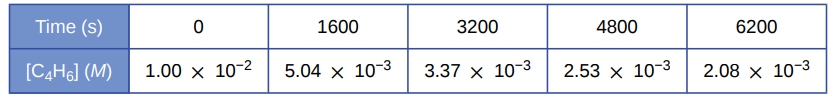# Problem: A study of the rate of dimerization of C4H6 gave the data shown in the table: 2 C4H6 ⟶ C8H12(b) Estimate the instantaneous rate of dimerization at 3200 s from a graph of time versus [C4H6]. What are the units of this rate?

###### FREE Expert Solution
83% (376 ratings)###### Problem Details

A study of the rate of dimerization of C4H6 gave the data shown in the table: 2 C4H6 ⟶ C8H12(b) Estimate the instantaneous rate of dimerization at 3200 s from a graph of time versus [C4H6]. What are the units of this rate?

Frequently Asked Questions

What scientific concept do you need to know in order to solve this problem?

Our tutors have indicated that to solve this problem you will need to apply the Instantaneous Rate of Change concept. If you need more Instantaneous Rate of Change practice, you can also practice Instantaneous Rate of Change practice problems.

What professor is this problem relevant for?

Based on our data, we think this problem is relevant for Professor Webb's class at ISU.

What textbook is this problem found in?

Our data indicates that this problem or a close variation was asked in Chemistry - OpenStax 2015th Edition. You can also practice Chemistry - OpenStax 2015th Edition practice problems.